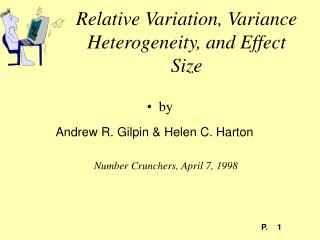# Relative Variation, Variance Heterogeneity, and Effect Size - PowerPoint PPT PresentationDownload PresentationRelative Variation, Variance Heterogeneity, and Effect Size

Relative Variation, Variance Heterogeneity, and Effect Size
Download Presentation## Relative Variation, Variance Heterogeneity, and Effect Size

- - - - - - - - - - - - - - - - - - - - - - - - - - - E N D - - - - - - - - - - - - - - - - - - - - - - - - - - -
##### Presentation Transcript

1. by Relative Variation, Variance Heterogeneity, and Effect Size Andrew R. Gilpin & Helen C. Harton Number Crunchers, April 7, 1998

2. Homogeneity of Variance as Assumption for Tests on Means Robustness of t, F Unequal n’s, non-normal data are troublesome

3. Variance as Dependent Variable Selection bias Differential influences between groups Learning Attitudinal shifts

4. Tests on Homogeneity of Variance Fisher’s F Levene’s ANOVA procedure (ANOVA on transformed scores) Miscellaneous other approaches Box Cochran Hartley O’Brien

5. Experimental Effect Size Cohen’s d Glass’s g Hedges’ h Pooled Variance Issue

6. Relative Variation Pearson’s Coefficient of Variation Means are often proportional to standard deviations Psychophysics research (Weber/Fechner Law) Physical size

7. Homogeneity of Relative Variation as a Null Hypothesis

8. Implications of Homogeneity of Relative Variation for h vs. g Pooled variance estimate based on smaller variance (and mean) will underestimate actual variance; pooled variance estimate based on larger variance (and mean) will overestimate actual variance. Distorted pooled variance will cause h to depart from g

9. Simulation Design 10,000 simulated experiments per cell 9 Populations (normal, 8 real radically non-normal) 9 Sample sizes (5,5), (25,25), (100,100), (5,25), (5,100), (25,100), (25,5), (100,5), (100,25) 3 Coefficient of Variation (V=.1, V=.2, V=.3) 6 Nominal g sizes: 0.0, 0.5, 1.0, 1.5, 2.0, 2.5

10. Simulation: Dependent variables Mean observed h Proportion (of 10,000) significant for =.05 Fisher’s F for variance heterogeneity Levene’s F (t) for variance heterogeneity

11. Observed h (100,100, Normal Population) Mean h Observed Nominal g

12. Power Curve for Levene’s Test (100,100, Normal Population) Proportion Significant Nominal g

13. Projected Sample Sizes Are Distorted Noncentrality parameter for independent-groups, equal N t-test For power (1-) = .80, =2.80 and N=15.68/d2 Estimated distortion from Normal population, (25,25)

14. Comparison of Estimated Sample Sizes Assumes N1=N2=N, Power=.80

15. Variance heterogeneity is implied by homogeneity of relative variation Use g rather than h if means are related to standard deviations General Conclusions

16. Suggestions, Anyone? How common is variance heterogeneity? How common is proportionality of means and standard deviations? Other?